# How to Calculate and Solve for Final Velocity | Motion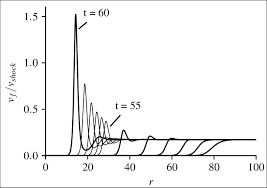The image above represents final velocity.

To compute for final velocity, three essential parameters are needed and these parameters are initial velocity (u), acceleration (a) and distance covered (S).

The formula for calculating final velocity:

v = √(u² + 2as)

Where;

v = Final Velocity
u = Initial Velocity
a = Acceleration
s = Distance Covered

Let’s solve an example;
Find the final velocity when the initial velocity is 13, acceleration is 9.8 and the distance covered is 32.

This implies that;

u = Initial Velocity = 13
a = Acceleration = 9.8
s = Distance Covered = 32

v = √(u² + 2as)
v = √(13² + (2 x 9.8 x 32))
v = √(169 + (627.2))
v = √(796.2)
v = 28.21

Therefore, the final velocity is 28.21 m/s.

Calculating the Initial Velocity when the Final Velocity, the Acceleration and the Distance Covered is Given.

u = √v² – 2as

Where;

u = Initial Velocity
v = Final Velocity
a = Acceleration
s = Distance Covered

Let’s solve an example;
Find the initial velocity when the final velocity is 30, the acceleration is 8 and the distance covered is 11.

This implies that;

v = Final Velocity = 30
a = Acceleration = 8
s = Distance Covered = 11

u = √v² – 2as
u = √30² – 2(8 x 11)
u = √900 – 2(88)
u = √900 – 176
u = √724
u = 26.90

Therefore, the initial velocity is 26.90 m/s.

Calculating the Acceleration when the Final Velocity, the Initial Velocity and the Distance Covered is Given.

a = v2 – u2 / 2s

Where;

a = Acceleration
v = Final Velocity
u = Initial Velocity
s = Distance Covered

Let’s solve an example;
Find the acceleration when the final velocity is 10, initial velocity is 8 and the distance covered is 7.

This implies that;

v = Final Velocity = 10
u = Initial Velocity = 8
s = Distance Covered = 7

a = v2 – u2 / 2s
a = 102 – 82 / 2(7)
a = 100 – 64 / 14
a = 36 / 14
a = 2.57

Therefore, the acceleration is 2.57.

Calculating the Distance Covered when the Final Velocity, Initial Velocity and Acceleration is Given.

s = v2 – u2 / 2a

Where;

s = Distance Covered
v = Final Velocity
u = Initial Velocity
a = Acceleration

Let’s solve an example;
Given that the final velocity is 20 and the acceleration is 10 and the initial velocity is 14. Find the distance covered?

This implies that;

v = Final Velocity = 20
u = Initial Velocity = 14
a = Acceleration = 10

s = v2 – u2 / 2a
s = 202 – 142 / 2(10)
s = 400 – 196 / 20
s = 204 / 20
s = 10.2

Therefore, the distance covered is 10.2 m.

Nickzom Calculator – The Calculator Encyclopedia is capable of calculating the final velocity.

To get the answer and workings of the final velocity using the Nickzom Calculator – The Calculator Encyclopedia. First, you need to obtain the app.

You can get this app via any of these means:

To get access to the professional version via web, you need to register and subscribe for NGN 1,500 per annum to have utter access to all functionalities.
You can also try the demo version via https://www.nickzom.org/calculator

Apple (Paid) – https://itunes.apple.com/us/app/nickzom-calculator/id1331162702?mt=8
Once, you have obtained the calculator encyclopedia app, proceed to the Calculator Map, then click on Motion under Physics.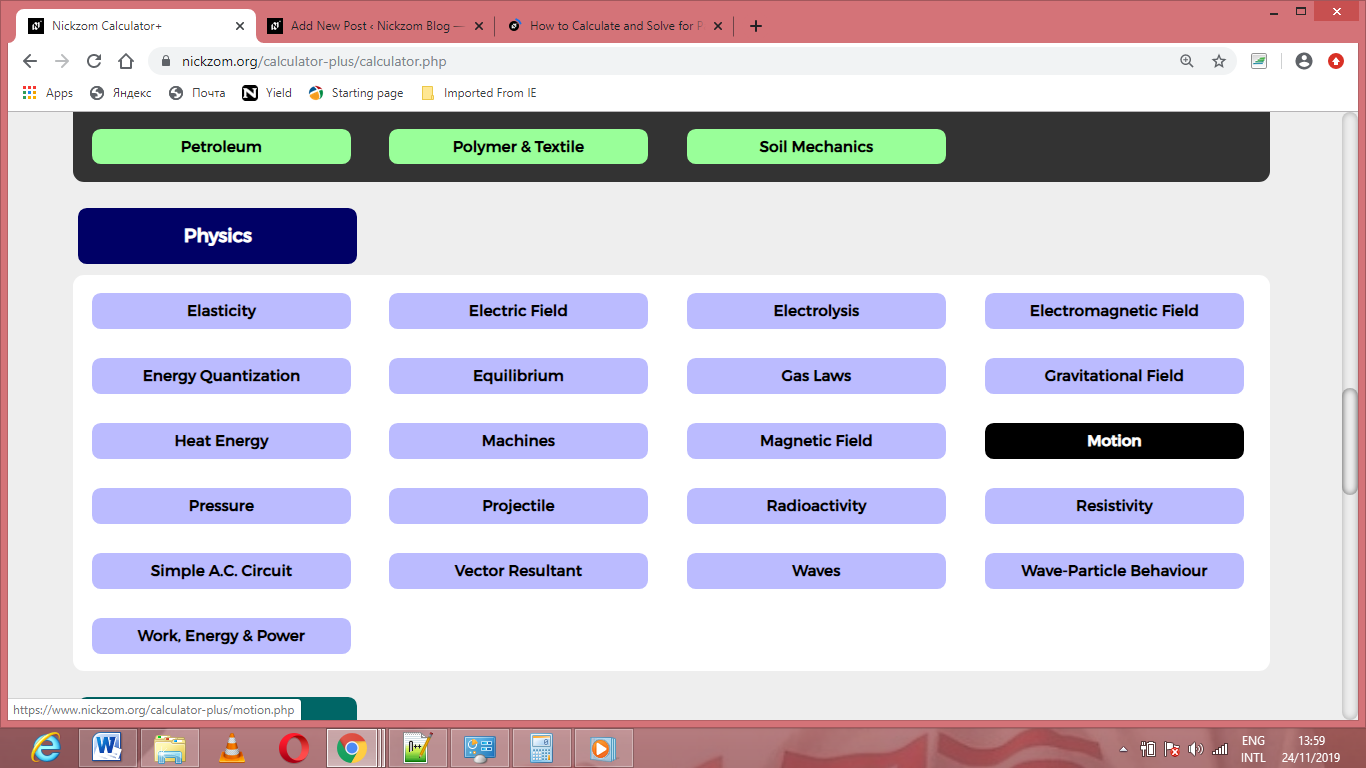Now, Click on Final Velocity under Motion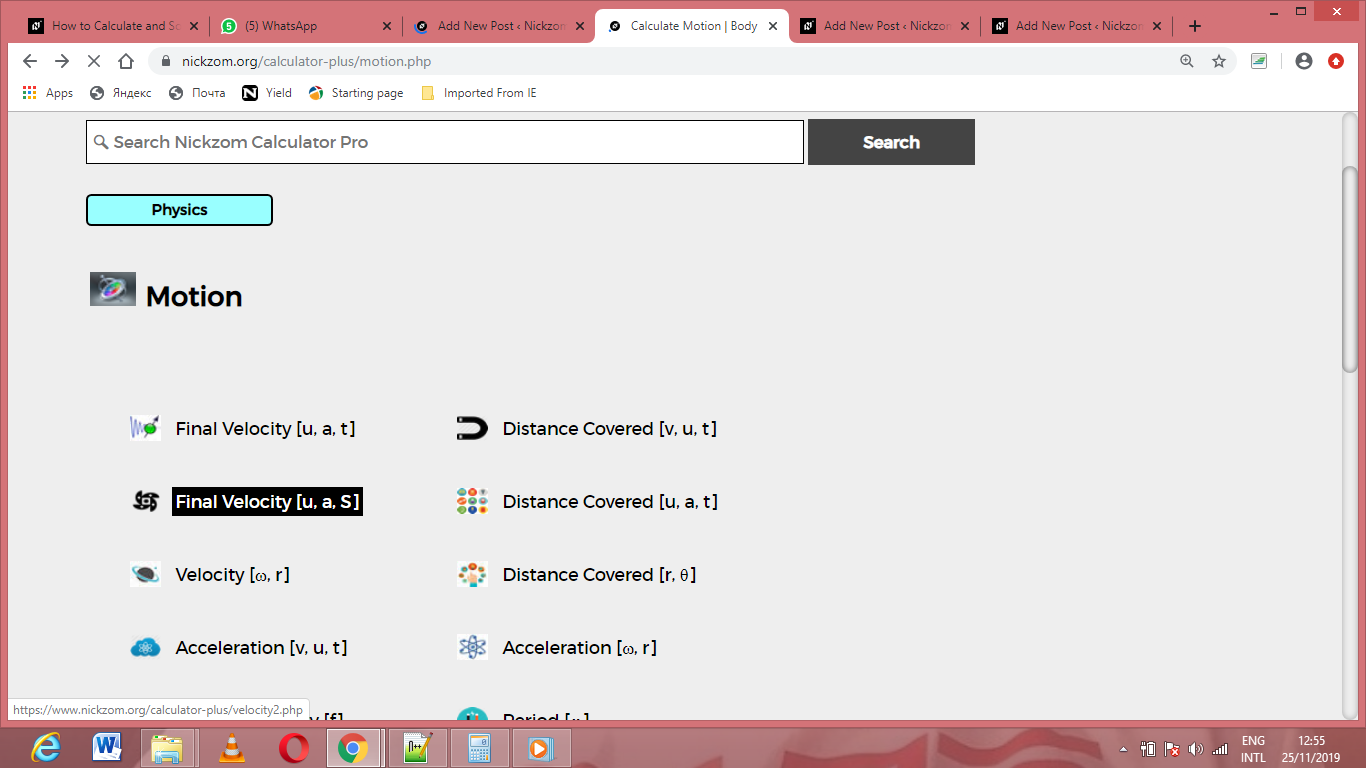The screenshot below displays the page or activity to enter your values, to get the answer for the final velocity according to the respective parameters which are the initial velocity (u), acceleration (a) and distance covered (s).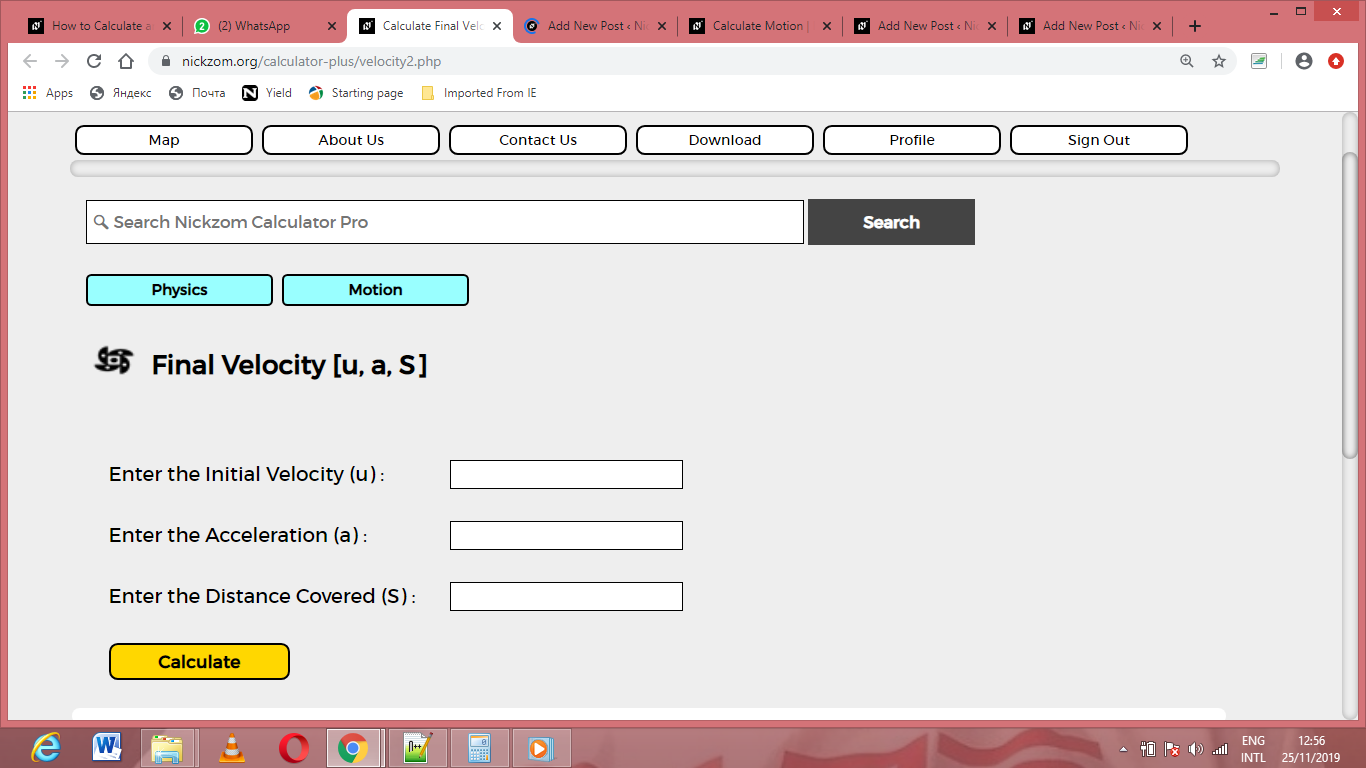Now, enter the values appropriately and accordingly for the parameters as required by the initial velocity (u) is 13, acceleration (a) is 9.8 and distance covered (s) is 32.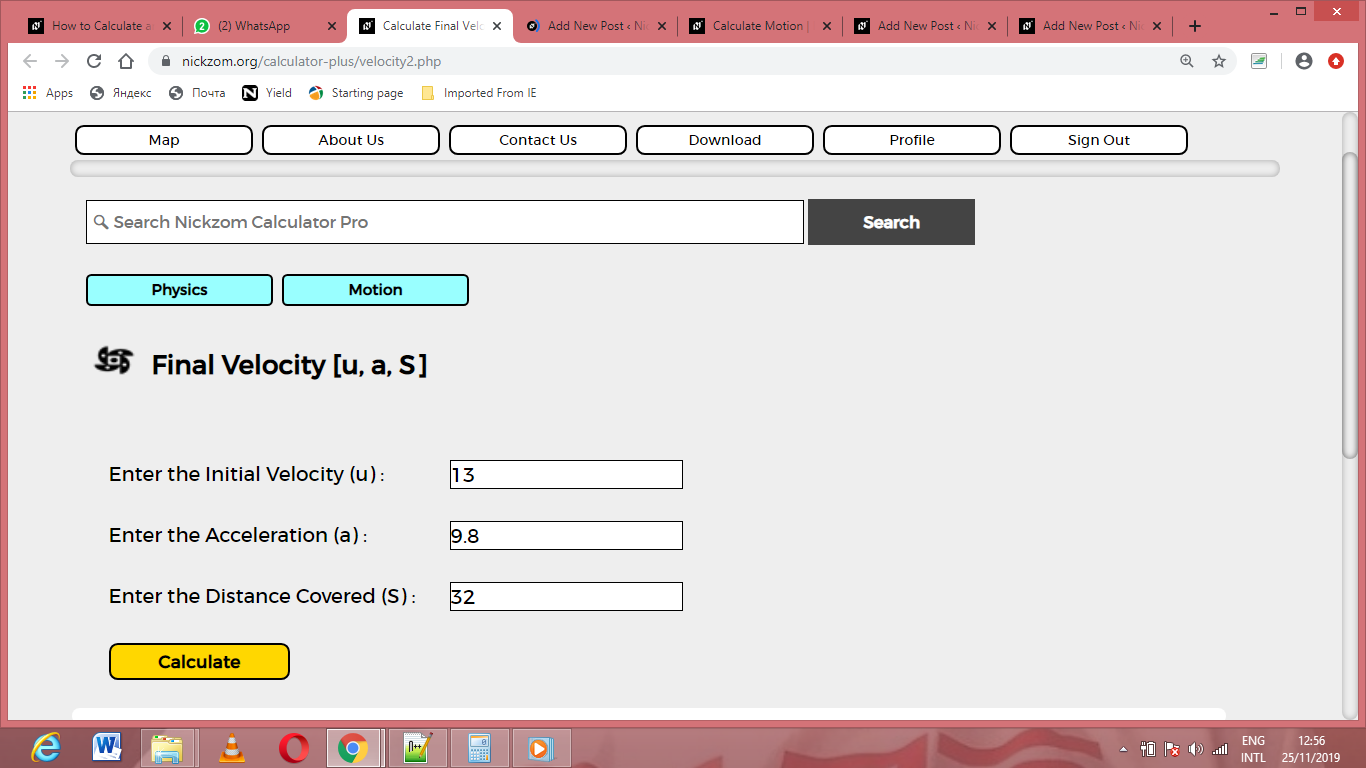Finally, Click on Calculate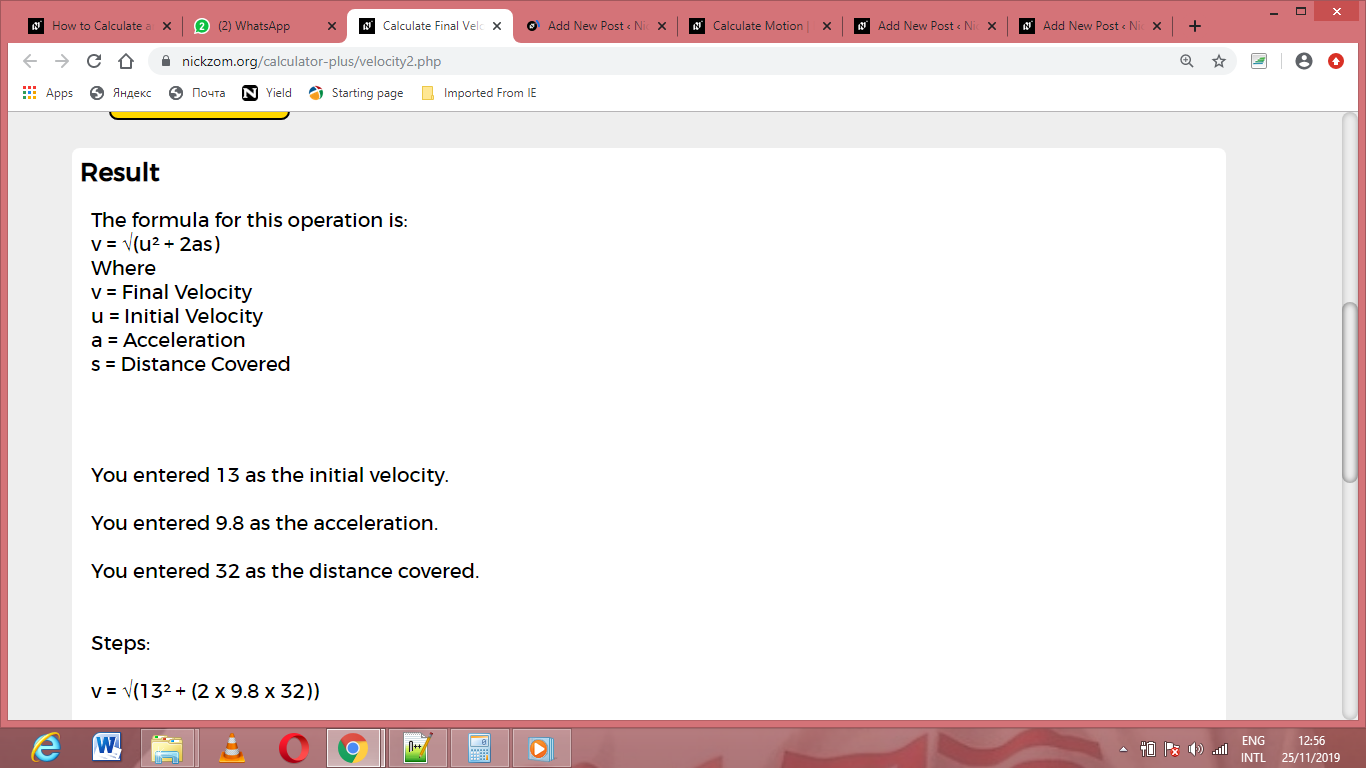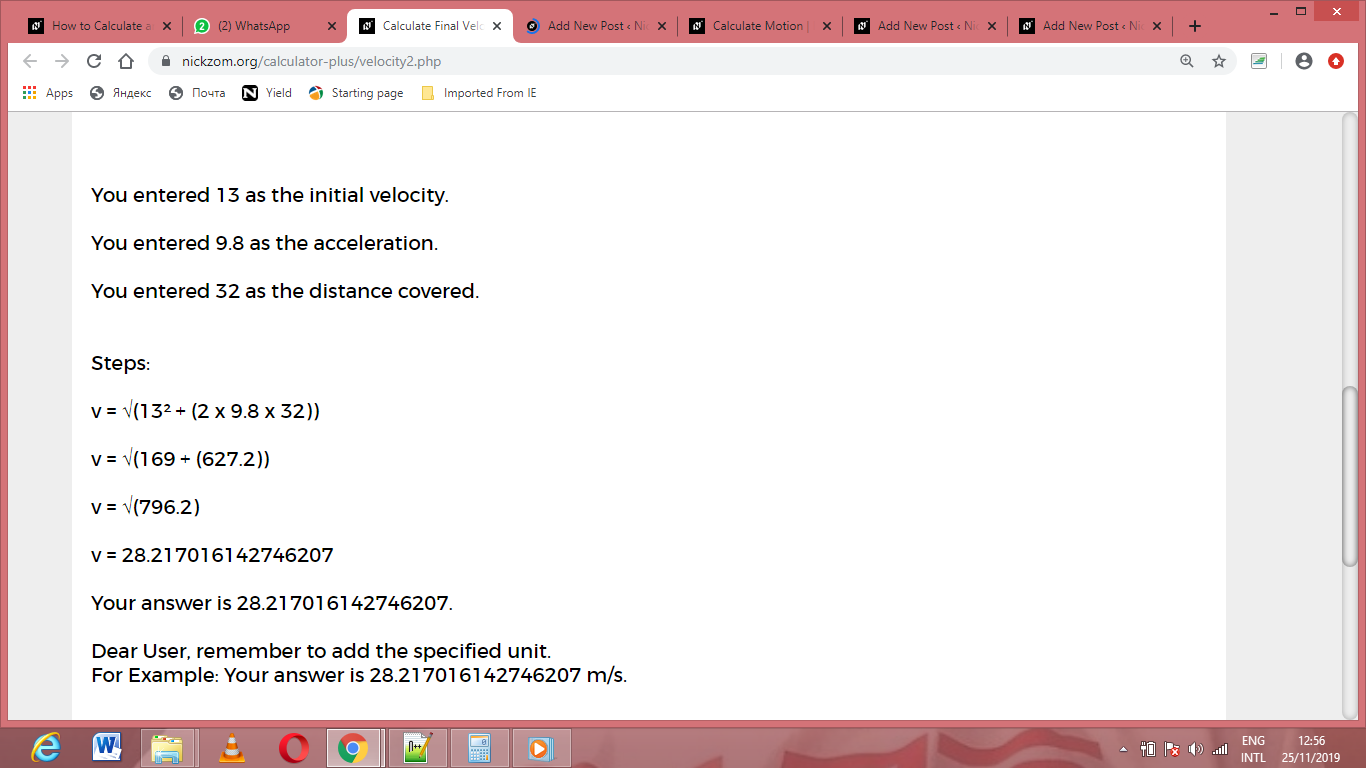As you can see from the screenshot above, Nickzom Calculator– The Calculator Encyclopedia solves for the final velocity and presents the formula, workings and steps too.